Equation of A Plane In The Normal Form

The equation of a plane in the normal form can be determined if normal to the plane as well as the distance of the plane from the origin is known. In this section, we will discuss the equation of a plane in the vector as well as Cartesian form.

Equation of a Plane in the Normal Form

The vector form of the equation of a plane in normal form is given by: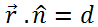Where $\vec{r}$ is the position vector of a point in the plane, n is the unit normal vector along the normal joining the origin to the plane and d is the perpendicular distance of the plane from the origin.

Let us say that P (x, y, z) is any point on the plane and O is the origin. Then, we have,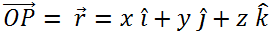Now we take the direction cosines of $\hat{n}$ as l, m and n. So we have,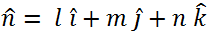From the equation $\vec{r}.\hat{n}$ = d we get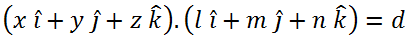Thus, the Cartesian form of the equation of a plane in normal form is given by:

lx + my + nz = d

Example

Let us take up an example to understand the equation of a plane in the normal form.

Problem: A plane is at a distance of $\frac{9}{\sqrt{38}}$ from the origin O. From the origin, its normal vector is given by 5$\hat{i}$ + 3$\hat{j}$ – 2$\hat{k}$.

What is the vector equation for the plane?

Solution: Let the normal vector be: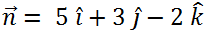We now find the unit vector for the normal vector. It can be given by: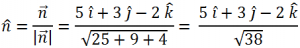So, the required equation of the plane can be given by substituting it in the vector equation: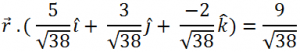We discussed an example for the equation of a plane in the normal form. To solve more examples and learn the concept in details by watching vibrant video lessons on them, download Byju’s The Learning App.